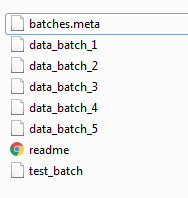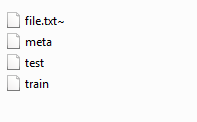### How to Load, Pre-process and Visualize CIFAR-10 and CIFAR -100 datasets in Python

In this post we discuss how to download the CIFAR-10 and CIFAR-100 dataset, how to read/ load these datasets. We do all preprocessing like reshape and Transpose the dataset before actually visualizing the images. The CIFAR-10 and CIFAR-100 images were collected by Alex Krizhevsky, Vinod Nair, and Geoffrey Hinton. The CIFAR-10 dataset consists of 60,000 color images of 32x32 size. The dataset has 10 classes, each class having 6,000 images. The dataset is divided in to two group training and testing images: 50,000 training images, 10,000 testing images. CIFAR-100 dataset also consists of 60,000 color images of 32x32 size. It has 100 classes, each contains 600 images. Per class, there are 500 trading images and 100 testing images.CIFAR-10image source: https://www.cs.toronto.edu/~kriz/cifar.html

Download the dataset from above link and unzip the file. For CIFAR-10, we get 5 training data batches: 'data_batch_1 -  'data_batch_5'  files, a test data batch 'test_batch' file and a ‘batch.meta’ file. For CIFAR-100 we get a ‘train’, ‘test’ and a ‘meta’ file. Eachof these files is a Python "pickled" object produced with cPickle. To open these files we use bellow Python3 routine.CIFAR-10CIFAR-100

#### Python 3 routine to open the file.

`def unpickle(file):    import pickle    with open(file, 'rb') as fo:        dict = pickle.load(fo, encoding='latin1')    return dict`

We use the above Python 3 function to open the files.

### CIFAR-10 Dataset

We load 'data_batch_1' data file using unpickle() method.

`file = r'D:\Binary Study\Datasets\cifar-10-python\cifar-10-batches-py\data_batch_1'data_batch_1 = unpickle(file)`

### Preprocessing CIFAR-10 Dataset

`print(type(data_batch_1))`

Output:

`<class 'dict'>`

Our training data ‘data_batch_1’ is in form of dictionary. Let’s look what are the keys in the dictionary.

`print(data_batch_1.keys())`

Output:

`dict_keys(['batch_label', 'labels', 'data', 'filenames'])`

There are four keys: batch_lebel, labels, data and filenames. Let’s look these four levels’ class types.

`for item in data_batch_1:    print(item, type(data_batch_1[item]))`

Output:

```batch_label <class 'str'>
labels <class 'list'>
data <class 'numpy.ndarray'>
filenames <class 'list'>```

We are more more interested in data na d labels. Let’s have look on labels and data.

`print("Labels:", set(data_batch_1['labels']))`

Output:

`Labels: {0, 1, 2, 3, 4, 5, 6, 7, 8, 9}`

We have 10 labels 0-9.

`X_train = data_batch_1['data']X_train`

Output:

```array([[ 59,  43,  50, ..., 140,  84,  72],
[154, 126, 105, ..., 139, 142, 144],
[255, 253, 253, ...,  83,  83,  84],
...,
[ 71,  60,  74, ...,  68,  69,  68],
[250, 254, 211, ..., 215, 255, 254],
[ 62,  61,  60, ..., 130, 130, 131]], dtype=uint8)```

Our image data is a numpy ndarray.

`X_train.shape`

Output:

`(10000, 3072)`

The whole data_batch_1 has 10,000 images. And each image is a 1-D array having 3,072 entries. First 1024 entries for Red, the next 1024 entries for Green and last 1024 entries for Blue channels. To visualise the images we have to change the shape of image as (32,32,3).

For the image label names, we load meta file ‘ batches.meta’ using same unpickle() function.

`meta_file = r'D:\Binary Study\Datasets\cifar-10-python\cifar-10-batches-py\batches.meta'meta_data = unpickle(meta_file)`

`print(type(meta_data))print(meta_data.keys()) `

Output:

```<class 'dict'>
dict_keys(['num_cases_per_batch', 'label_names', 'num_vis'])```

The meta file is a dictionary and has three keys. We are more interested here for ‘label_names’.

`print("Label Names:", meta_data['label_names'] )`

Output:

`Label Names: ['airplane', 'automobile', 'bird', 'cat', 'deer', 'dog', 'frog', 'horse', 'ship', 'truck']`

The ten label names are given above.

#### Reshape And Transpose a Single Image

Our image is a single dimension array of size 3072. First 1024 entries of the array are of Red channel, next 1024 entries are of Green channel, and last 1024 entries are of Blue channel. Total 3072 entries are of three RGB channels.

• First we reshape the image/ array into (3,32,32) using image.reshape(3,32,32). (32,32) for 32x32 =1024 entries and 3 for RGB channels.

`image = data_batch_1[b'data']image = image.reshape(3,32,32)print(image.shape)`

Output:

`(3, 32, 32)`

• Next we transpose the reshaped image to get the image of shape (32,32,3). We use image.transpose(1,2,0).

`image = image.transpose(1,2,0)print(image.shape)`

Output:

`(32, 32, 3)`

Now our image in the right format for display using Matplotlib.

### Visualization

#### Visualizing Single Image From CIFAR-10 Dataset

We first visualize a single image using Matplotlib. We use plt.imshow() to display the image. Let’s visualize first image. Look below Python3 code.

#### Output:CIFAR-10 First Image with Label Name: Frog

#### Visualizing Multiple Images From CIFAR-10 Dataset

We visualize some randomly selected images from the training data batch.

#### `# Python 3 program to visualize 4th imageimport matplotlib.pyplot as pltimport numpy as np# take the images data from batch dataimages = data_batch_1['data']# reshape and transpose the imagesimages = images.reshape(len(images),3,32,32).transpose(0,2,3,1)# take labels of the images labels = data_batch_1['labels']# label names of the imageslabel_names = meta_data['label_names']# dispaly random images# define row and column of figurerows, columns = 5, 5# take random image idex idimageId = np.random.randint(0, len(images), rows * columns)# take images for above random image idsimages = images[imageId]# take labels for these images onlylabels = [labels[i] for i in imageId]# define figurefig=plt.figure(figsize=(10, 10))# visualize these random imagesfor i in range(1, columns*rows +1):    fig.add_subplot(rows, columns, i)    plt.imshow(images[i-1])    plt.xticks([])    plt.yticks([])    plt.title("{}"          .format(label_names[labels[i-1]]))plt.show()`

Output:Random Images

You may be interested in the following post related CIFAR 10 dataset.

### CIFAR-100 Dataset

In CIFAR-100, the images are categorised in two different categories. The images are first categorised at coarse label. There is 20 classes at coarse label. Each category is further sub-categorised at fine labels. Each coarse class  has 5 fine classes. Hence total 100 classes in CIFAR-100 dataset.

We load train data file using unpickle() method.

`file = r'D:\Binary Study\Datasets\cifar-100-python\train'train_data = unpickle(file)`

### Pre-processing CIFAR-100 Dataset

`print(type(train_data))`

Output:

`<class 'dict'>`

`print(train_data.keys())`

Output:

`dict_keys(['filenames', 'batch_label', 'fine_labels', 'coarse_labels', 'data'])`

The train data is a dictionary having 5 keys. Let’s look what are the class type of these key.

`for item in train_data:    print(item, type(train_data[item]))`

Output:

```filenames <class 'list'>
batch_label <class 'str'>
fine_labels <class 'list'>
coarse_labels <class 'list'>
data <class 'numpy.ndarray'>```

Here we are more interested in ‘data’, ‘coarse_labels’, and ‘fine_labels’. Let’s look at the coarse and fine labels.

`print("Fine Labels:", set(train_data['fine_labels']))print("Coarse Labels:", set(train_data['coarse_labels']))`

Output:

`Fine Labels: {0, 1, 2, 3, 4, 5, 6, 7, 8, 9, 10, 11, 12, 13, 14, 15, 16, 17, 18, 19, 20, 21, 22, 23, 24, 25, 26, 27, 28, 29, 30, 31, 32, 33, 34, 35, 36, 37, 38, 39, 40, 41, 42, 43, 44, 45, 46, 47, 48, 49, 50, 51, 52, 53, 54, 55, 56, 57, 58, 59, 60, 61, 62, 63, 64, 65, 66, 67, 68, 69, 70, 71, 72, 73, 74, 75, 76, 77, 78, 79, 80, 81, 82, 83, 84, 85, 86, 87, 88, 89, 90, 91, 92, 93, 94, 95, 96, 97, 98, 99}Coarse Labels: {0, 1, 2, 3, 4, 5, 6, 7, 8, 9, 10, 11, 12, 13, 14, 15, 16, 17, 18, 19}`

The are 100 fine labels 0-99, and 20 coarse labels 0-19.

Let’s have a look at the data.

`X_train = train_data['data']X_train`

Output:

```array([[255, 255, 255, ...,  10,  59,  79],
[255, 253, 253, ..., 253, 253, 255],
[250, 248, 247, ..., 194, 207, 228],
...,
[248, 240, 236, ..., 180, 174, 205],
[156, 151, 151, ..., 114, 107, 126],
[ 31,  30,  31, ...,  72,  69,  67]], dtype=uint8)```

Our data is a numpy ndarray.

`X_train.shape`

Output:

`(50000, 3072)`

Here the training data has 50,000 images as numpy arrays. Each image is a single dimension array having 3072 values. As we have seen in the case of CIFAR-10 we proceed same for dataset reshape and transpose to make images in suitable format for visualisation purpose in Matplotlib.

We load meta file for the coarse and fine label names.

`meta_file = r'D:\Binary Study\Datasets\cifar-100-python\meta'meta_data = unpickle(meta_file)`

`print(type(meta_data))print(meta_data.keys())`

Output:

`<class 'dict'>dict_keys(['fine_label_names', 'coarse_label_names'])`

Print all Labels

`print("Fine Label Names:", meta_data['fine_label_names'] )`

Output:

`Fine Label Names: ['apple', 'aquarium_fish', 'baby', 'bear', 'beaver', 'bed', 'bee', 'beetle', 'bicycle', 'bottle', 'bowl', 'boy', 'bridge', 'bus', 'butterfly', 'camel', 'can', 'castle', 'caterpillar', 'cattle', 'chair', 'chimpanzee', 'clock', 'cloud', 'cockroach', 'couch', 'crab', 'crocodile', 'cup', 'dinosaur', 'dolphin', 'elephant', 'flatfish', 'forest', 'fox', 'girl', 'hamster', 'house', 'kangaroo', 'keyboard', 'lamp', 'lawn_mower', 'leopard', 'lion', 'lizard', 'lobster', 'man', 'maple_tree', 'motorcycle', 'mountain', 'mouse', 'mushroom', 'oak_tree', 'orange', 'orchid', 'otter', 'palm_tree', 'pear', 'pickup_truck', 'pine_tree', 'plain', 'plate', 'poppy', 'porcupine', 'possum', 'rabbit', 'raccoon', 'ray', 'road', 'rocket', 'rose', 'sea', 'seal', 'shark', 'shrew', 'skunk', 'skyscraper', 'snail', 'snake', 'spider', 'squirrel', 'streetcar', 'sunflower', 'sweet_pepper', 'table', 'tank', 'telephone', 'television', 'tiger', 'tractor', 'train', 'trout', 'tulip', 'turtle', 'wardrobe', 'whale', 'willow_tree', 'wolf', 'woman', 'worm']`

Print all Coarse Labels.

`print("Coarse Label Names:", meta_data['coarse_label_names'] )`

Output:

`Coarse Label Names: ['aquatic_mammals', 'fish', 'flowers', 'food_containers', 'fruit_and_vegetables', 'household_electrical_devices', 'household_furniture', 'insects', 'large_carnivores', 'large_man-made_outdoor_things', 'large_natural_outdoor_scenes', 'large_omnivores_and_herbivores', 'medium_mammals', 'non-insect_invertebrates', 'people', 'reptiles', 'small_mammals', 'trees', 'vehicles_1', 'vehicles_2']`

#### Reshape And Transpose CIFAR-100 Dataset

We reshape and transpose dataset as we did in the CIFAR-10.

`X_train = train_data['data']# Reshape the whole image dataX_train = X_train.reshape(len(X_train),3,32,32)# Transpose the whole dataX_train = X_train.transpose(0,2,3,1)`

### Visualization

#### Visualizing Single Image From CIFAR-100 Dataset

We visualize a single image using Matplotlib library. We display the actual coarse and fine label names of images.

`# Python 3 program to visualize 4th imageimport matplotlib.pyplot as plt# take 4th image from training dataimage = train_data['data']# reshape and transpose the imageimage = image.reshape(3,32,32).transpose(1,2,0)# take coarse and fine labels of the image c_label = train_data['coarse_labels']f_label = train_data['fine_labels']# take coarse and fine label names of the imagecoarse_name = meta_data['coarse_label_names'][c_label]fine_name = meta_data['fine_label_names'][f_label]# dispaly the imageplt.imshow(image)plt.title("Coarse Label Name:{} \n Fine Label Name:{}"          .format(coarse_name, fine_name))`

Output:image with coarse and fine label names

#### Visualizing Multiple Images From CIFAR-100 Dataset

Let’s visualize some random images from the dataset with actual coarse and fine label names.

`# Python 3 program to visualize 4th imageimport matplotlib.pyplot as pltimport numpy as np# take the images data from training dataimages = train_data['data']# reshape and transpose the imagesimages = images.reshape(len(images),3,32,32).transpose(0,2,3,1)# take coarse and fine labels of the images c_labels = train_data['coarse_labels']# print(c_labels)f_labels = train_data['fine_labels']# take coarse and fine label names of the imagescoarse_names = meta_data['coarse_label_names']fine_names = meta_data['fine_label_names']# dispaly random nine images# define row and column of figurerows, columns = 3, 3# take random image idex idimageId = np.random.randint(0, len(images), rows * columns)# take images for above random image idsimages = images[imageId]# take coarse and fine labels for these images onlyc_labels = [c_labels[i] for i in imageId]f_labels = [f_labels[j] for j in imageId]# define figurefig=plt.figure(figsize=(8, 10))# visualize these random imagesfor i in range(1, columns*rows +1):    fig.add_subplot(rows, columns, i)    plt.imshow(images[i-1])    plt.xticks([])    plt.yticks([])    plt.title("{} \n {}"          .format(coarse_names[c_labels[i-1]], fine_names[f_labels[i-1]]))plt.show()`

Output:Multiple Random Images

How To Posts